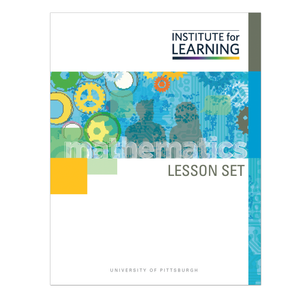# Understanding Congruence and Similarity (Grade 8)

Regular price \$6.25 \$0.00 Unit price per

In this lesson set, students will be introduced to congruence and similarity through their exploration of the reflection, rotation, translation, and dilation of figures in a plane. The first four tasks develop and solidify students’ intuitive understanding of reflection, rotation, and translation, and the next three tasks develop their intuitive understanding of dilation as it relates to similarity. In the final task, they combine all four of these motions to solidify their understanding of congruence and similarity.

Through engaging in the lesson set, students will:

• develop an intuitive understanding of rigid motion in terms of the movement of objects in the plane;
• develop an intuitive understanding of dilation in terms of expansion, or contraction, of an object with respect to a center of dilation;
• describe a sequence of rigid motions and dilations that will move one shape onto another in the plane; and
• develop an understanding of congruence and similarity and their connection to rigid motions and dilations.

Standards: 8.G.A.1, 8.G.A.1.A, 8.G.A.1.B, 8.G.A.1.C, 8.G.A.2, 8.G.A.4